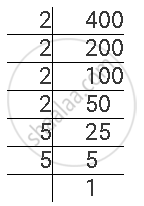Share

# Find the Square Roots of the Given Numbers by the Prime Factorisation Method. 400 - Mathematics

Course

#### Question

Find the square roots of the given numbers by the Prime Factorisation Method.

400

#### Solution

400 can be factorised as follows.400 = 2 × 2 × 2 × 2 × 5 × 5

∴ sqrt400  = 2 xx 2 xx 5 = 20

Is there an error in this question or solution?